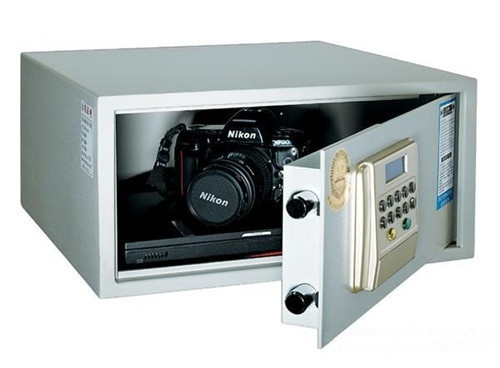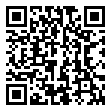|

# 保险柜怎么设置密码 机械和电子哪个保险柜好

随着现在的人对于秘密和隐私的不断重视，很多企业在给员工采购文件柜时，通常都会选择一些带有锁具的文件柜，而企业老总的文件柜通常都像电视里所看到的那样，选用那种高档的电子保险柜。但是有的时候可能对于电子保险柜的使用并不频繁，进而导致了我们哪一天突然要取出保险柜里的文件或是想存储资料时忘记了自己原先设定的保险柜密码。那么，保险柜怎么设置密码？机械和电子哪个保险柜好，下面就随小编一起看看吧。机械保险柜和电子保险柜哪个好？

目前常见的保险柜有电子锁、机械密码锁、刷卡式、指纹锁以及遥控密码锁等，而比较常见的还是机械锁和电子锁两种，两种锁各有利弊，下面简单的做一分析：

1、机械密码锁一般指转盘密码锁，安全性比较高，稳定、耐用，不需要电源。但是操作方法比较复杂、开启不方便，密码修改需要专业人员。

2、电子密码锁操作简单、容易开启，修改密码也比较简单随意，密码遗忘后还有备用钥匙可以打开更换密码，而且现在的电子锁只要是正规大厂家的产品稳性都不错，一般不容易坏。但是电子密码锁的安全性普遍没有机械锁的高。

电子保险柜

（1）、电子密码锁出厂设置的统一密码为：个人码“1234”，管理码：“123456”。

（2）、操作键板上“#”键为启动键，安装电池后密码锁自动扫描，扫描后屏显“ALLREADY”并进入睡眠状态，按“#”键可开户液晶屏及线路板进入工作状态。

（3）、操作键板上“#”键为清除键，用于清除输入的错误数字，每按一次清除一个数字。

（4）、每按一次数字键蜂鸣器响一声，液晶屏显示输入数字，表示数字已输入，任何键按下10秒，无后续操作，电脑板自动进入睡眠状态，液晶屏上将显示年、月、日和时、分及星期，按下“#”唤醒，将重新进入功能操作。

1、个人密码的设置与更改

先按“#”键唤醒黄屏，输入正确的1-8位数字的个人码后，按“#”键确认，蜂鸣器响两声，液晶屏显示“OPEN”且蓝屏亮表示密码正确，电磁铁吸合6秒，在7秒内按下“#”键确认，液晶屏显示“INTO”，表示初级密码输入成功，如果已设置了密码，则再进行的密码设置将只是对原有密码的更改。

2、管理码的设置与更改

按“#”键唤醒电脑板，此时黄屏亮，输入正确的1-8位管理码后，按“#”键确认，蜂鸣器响两声，液晶屏显示“OPEN”且蓝屏亮，表示密码正确。电磁铁吸合6秒，在7秒内按下“#”键进管理密码的修改状态，同时显示“—”，表示可设置管理密码，任意输入1—8位数字的密码后按“#”键确认，液晶屏显示“INTO”，表示管理密码输入成功。如果已设置了密码，则所进行的设置只是对原来密码的更改。

3、隐码功能

按“#”键唤醒黄屏，输入密码前，按“#”键一次，则所输入的密码不显示数字，开门后自动恢复不隐数字。机械保险柜

机械保险箱的常规开启方法(左79，右42，左16)：

把密码盘朝顺时针方向转动，使盘上的刻度值“79”对准盘上的基准点，然后继续朝顺时针方向转三圈或三圈以上，对准79；逆时针转过79，继续逆时针对准42；顺指针直接对准16.

规律：顺~逆~顺 ，3~2~1. 动作要慢，过了重来。

如何更改机械保险柜密码锁。

第一步，先拆下保险柜门里面挡板，把密码盘上的螺丝任意换一孔，现在的秘码就改变了。这时不要把门关上 密码已改变！！！

第二步，寻找新的密码，先用手在外面向右空转两周密码盘后，再看着柜门里面密码锁的最里面的“叶片”，现在用手继续在外面转动密码盘，直到叶片上的真正豁口对准和保险柜外面开锁扳手联动的导向柱（开启时导向柱是要插进这个豁口的），现在看一下密码盘上对着红点数字就是保险柜的新的第一组密码。第一组密码找到后，以它为起点先向左空转一圈后，和找第一组密码一样看着里面的中间“叶片”再向左转动密码盘，直至“叶片”上的真豁口对准导向柱时，看一下密码盘上对准红点的数字就是第二组密码。接下来再用手直接向右转动密码盘，看到里面最外面的“叶片”真豁口对准导向柱时，密码盘上对着红点的数字就是第三组密码。三组新密码找到后先别急于关门，在开门状态下反复试验几次后，确认新密码开启无误后再关保险柜门。

总结：关于保险柜怎么设置密码、机械和电子哪个保险柜好的相关信息就为大家介绍到这里了，希望这篇文章对大家有所帮助。如果大家还有什么不明白的地方可以在下方给小编留言哦，我们会尽快为您解答。

`声明：本文由入驻焦点开放平台的作者撰写，除焦点官方账号外，观点仅代表作者本人，不代表焦点立场错误信息举报电话： 400-099-0099，邮箱：jubao@vip.sohu.com，或点此进行意见反馈，或点此进行举报投诉。`A B C D E F G H J K L M N P Q R S T W X Y Z
A - B - C - D - E
• A
• 鞍山
• 安庆
• 安阳
• 安顺
• 安康
• 澳门
• B
• 北京
• 保定
• 包头
• 巴彦淖尔
• 本溪
• 蚌埠
• 亳州
• 滨州
• 北海
• 百色
• 巴中
• 毕节
• 保山
• 宝鸡
• 白银
• 巴州
• C
• 承德
• 沧州
• 长治
• 赤峰
• 朝阳
• 长春
• 常州
• 滁州
• 池州
• 长沙
• 常德
• 郴州
• 潮州
• 崇左
• 重庆
• 成都
• 楚雄
• 昌都
• 慈溪
• 常熟
• D
• 大同
• 大连
• 丹东
• 大庆
• 东营
• 德州
• 东莞
• 德阳
• 达州
• 大理
• 德宏
• 定西
• 儋州
• 东平
• E
• 鄂尔多斯
• 鄂州
• 恩施
F - G - H - I - J
• F
• 抚顺
• 阜新
• 阜阳
• 福州
• 抚州
• 佛山
• 防城港
• G
• 赣州
• 广州
• 桂林
• 贵港
• 广元
• 广安
• 贵阳
• 固原
• H
• 邯郸
• 衡水
• 呼和浩特
• 呼伦贝尔
• 葫芦岛
• 哈尔滨
• 黑河
• 淮安
• 杭州
• 湖州
• 合肥
• 淮南
• 淮北
• 黄山
• 菏泽
• 鹤壁
• 黄石
• 黄冈
• 衡阳
• 怀化
• 惠州
• 河源
• 贺州
• 河池
• 海口
• 红河
• 汉中
• 海东
• 怀来
• I
• J
• 晋中
• 锦州
• 吉林
• 鸡西
• 佳木斯
• 嘉兴
• 金华
• 景德镇
• 九江
• 吉安
• 济南
• 济宁
• 焦作
• 荆门
• 荆州
• 江门
• 揭阳
• 金昌
• 酒泉
• 嘉峪关
K - L - M - N - P
• K
• 开封
• 昆明
• 昆山
• L
• 廊坊
• 临汾
• 辽阳
• 连云港
• 丽水
• 六安
• 龙岩
• 莱芜
• 临沂
• 聊城
• 洛阳
• 漯河
• 娄底
• 柳州
• 来宾
• 泸州
• 乐山
• 六盘水
• 丽江
• 临沧
• 拉萨
• 林芝
• 兰州
• 陇南
• M
• 牡丹江
• 马鞍山
• 茂名
• 梅州
• 绵阳
• 眉山
• N
• 南京
• 南通
• 宁波
• 南平
• 宁德
• 南昌
• 南阳
• 南宁
• 内江
• 南充
• P
• 盘锦
• 莆田
• 平顶山
• 濮阳
• 攀枝花
• 普洱
• 平凉
Q - R - S - T - W
• Q
• 秦皇岛
• 齐齐哈尔
• 衢州
• 泉州
• 青岛
• 清远
• 钦州
• 黔南
• 曲靖
• 庆阳
• R
• 日照
• 日喀则
• S
• 石家庄
• 沈阳
• 双鸭山
• 绥化
• 上海
• 苏州
• 宿迁
• 绍兴
• 宿州
• 三明
• 上饶
• 三门峡
• 商丘
• 十堰
• 随州
• 邵阳
• 韶关
• 深圳
• 汕头
• 汕尾
• 三亚
• 三沙
• 遂宁
• 山南
• 商洛
• 石嘴山
• T
• 天津
• 唐山
• 太原
• 通辽
• 铁岭
• 泰州
• 台州
• 铜陵
• 泰安
• 铜仁
• 铜川
• 天水
• 天门
• W
• 乌海
• 乌兰察布
• 无锡
• 温州
• 芜湖
• 潍坊
• 威海
• 武汉
• 梧州
• 渭南
• 武威
• 吴忠
• 乌鲁木齐
X - Y - Z
• X
• 邢台
• 徐州
• 宣城
• 厦门
• 新乡
• 许昌
• 信阳
• 襄阳
• 孝感
• 咸宁
• 湘潭
• 湘西
• 西双版纳
• 西安
• 咸阳
• 西宁
• 仙桃
• 西昌
• Y
• 运城
• 营口
• 盐城
• 扬州
• 鹰潭
• 宜春
• 烟台
• 宜昌
• 岳阳
• 益阳
• 永州
• 阳江
• 云浮
• 玉林
• 宜宾
• 雅安
• 玉溪
• 延安
• 榆林
• 银川
• Z
• 张家口
• 镇江
• 舟山
• 漳州
• 淄博
• 枣庄
• 郑州
• 周口
• 驻马店
• 株洲
• 张家界
• 珠海
• 湛江
• 肇庆
• 中山
• 自贡
• 资阳
• 遵义
• 昭通
• 张掖
• 中卫

1室1厅1厨1卫1阳台

1
2
3
4
5

0
1
2

1

1

0
1
2
3报名成功，资料已提交审核A B C D E F G H J K L M N P Q R S T W X Y Z
A - B - C - D - E
• A
• 鞍山
• 安庆
• 安阳
• 安顺
• 安康
• 澳门
• B
• 北京
• 保定
• 包头
• 巴彦淖尔
• 本溪
• 蚌埠
• 亳州
• 滨州
• 北海
• 百色
• 巴中
• 毕节
• 保山
• 宝鸡
• 白银
• 巴州
• C
• 承德
• 沧州
• 长治
• 赤峰
• 朝阳
• 长春
• 常州
• 滁州
• 池州
• 长沙
• 常德
• 郴州
• 潮州
• 崇左
• 重庆
• 成都
• 楚雄
• 昌都
• 慈溪
• 常熟
• D
• 大同
• 大连
• 丹东
• 大庆
• 东营
• 德州
• 东莞
• 德阳
• 达州
• 大理
• 德宏
• 定西
• 儋州
• 东平
• E
• 鄂尔多斯
• 鄂州
• 恩施
F - G - H - I - J
• F
• 抚顺
• 阜新
• 阜阳
• 福州
• 抚州
• 佛山
• 防城港
• G
• 赣州
• 广州
• 桂林
• 贵港
• 广元
• 广安
• 贵阳
• 固原
• H
• 邯郸
• 衡水
• 呼和浩特
• 呼伦贝尔
• 葫芦岛
• 哈尔滨
• 黑河
• 淮安
• 杭州
• 湖州
• 合肥
• 淮南
• 淮北
• 黄山
• 菏泽
• 鹤壁
• 黄石
• 黄冈
• 衡阳
• 怀化
• 惠州
• 河源
• 贺州
• 河池
• 海口
• 红河
• 汉中
• 海东
• 怀来
• I
• J
• 晋中
• 锦州
• 吉林
• 鸡西
• 佳木斯
• 嘉兴
• 金华
• 景德镇
• 九江
• 吉安
• 济南
• 济宁
• 焦作
• 荆门
• 荆州
• 江门
• 揭阳
• 金昌
• 酒泉
• 嘉峪关
K - L - M - N - P
• K
• 开封
• 昆明
• 昆山
• L
• 廊坊
• 临汾
• 辽阳
• 连云港
• 丽水
• 六安
• 龙岩
• 莱芜
• 临沂
• 聊城
• 洛阳
• 漯河
• 娄底
• 柳州
• 来宾
• 泸州
• 乐山
• 六盘水
• 丽江
• 临沧
• 拉萨
• 林芝
• 兰州
• 陇南
• M
• 牡丹江
• 马鞍山
• 茂名
• 梅州
• 绵阳
• 眉山
• N
• 南京
• 南通
• 宁波
• 南平
• 宁德
• 南昌
• 南阳
• 南宁
• 内江
• 南充
• P
• 盘锦
• 莆田
• 平顶山
• 濮阳
• 攀枝花
• 普洱
• 平凉
Q - R - S - T - W
• Q
• 秦皇岛
• 齐齐哈尔
• 衢州
• 泉州
• 青岛
• 清远
• 钦州
• 黔南
• 曲靖
• 庆阳
• R
• 日照
• 日喀则
• S
• 石家庄
• 沈阳
• 双鸭山
• 绥化
• 上海
• 苏州
• 宿迁
• 绍兴
• 宿州
• 三明
• 上饶
• 三门峡
• 商丘
• 十堰
• 随州
• 邵阳
• 韶关
• 深圳
• 汕头
• 汕尾
• 三亚
• 三沙
• 遂宁
• 山南
• 商洛
• 石嘴山
• T
• 天津
• 唐山
• 太原
• 通辽
• 铁岭
• 泰州
• 台州
• 铜陵
• 泰安
• 铜仁
• 铜川
• 天水
• 天门
• W
• 乌海
• 乌兰察布
• 无锡
• 温州
• 芜湖
• 潍坊
• 威海
• 武汉
• 梧州
• 渭南
• 武威
• 吴忠
• 乌鲁木齐
X - Y - Z
• X
• 邢台
• 徐州
• 宣城
• 厦门
• 新乡
• 许昌
• 信阳
• 襄阳
• 孝感
• 咸宁
• 湘潭
• 湘西
• 西双版纳
• 西安
• 咸阳
• 西宁
• 仙桃
• 西昌
• Y
• 运城
• 营口
• 盐城
• 扬州
• 鹰潭
• 宜春
• 烟台
• 宜昌
• 岳阳
• 益阳
• 永州
• 阳江
• 云浮
• 玉林
• 宜宾
• 雅安
• 玉溪
• 延安
• 榆林
• 银川
• Z
• 张家口
• 镇江
• 舟山
• 漳州
• 淄博
• 枣庄
• 郑州
• 周口
• 驻马店
• 株洲
• 张家界
• 珠海
• 湛江
• 肇庆
• 中山
• 自贡
• 资阳
• 遵义
• 昭通
• 张掖
• 中卫• 手机• 分享
• 设计
免费设计
• 计算器
装修计算器
• 入驻
合作入驻
• 联系
联系我们
• 置顶
返回顶部